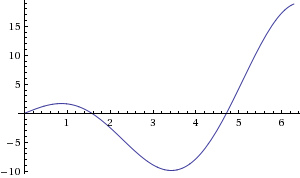Home > APCALC > Chapter 1 > Lesson 1.4.2 > Problem1-153

1-153.

For $f(x) = 3x \cos(x)$, approximate the area under the curve for $0≤x≤\frac { \pi } { 2 }$ using two different methods. If the actual area is approximately $1.712$ square units, which of your methods was most accurate? Analyze why that particular method was more accurate.Answers vary, but be sure to take into account the width of the rectangles and/or trapezoids.